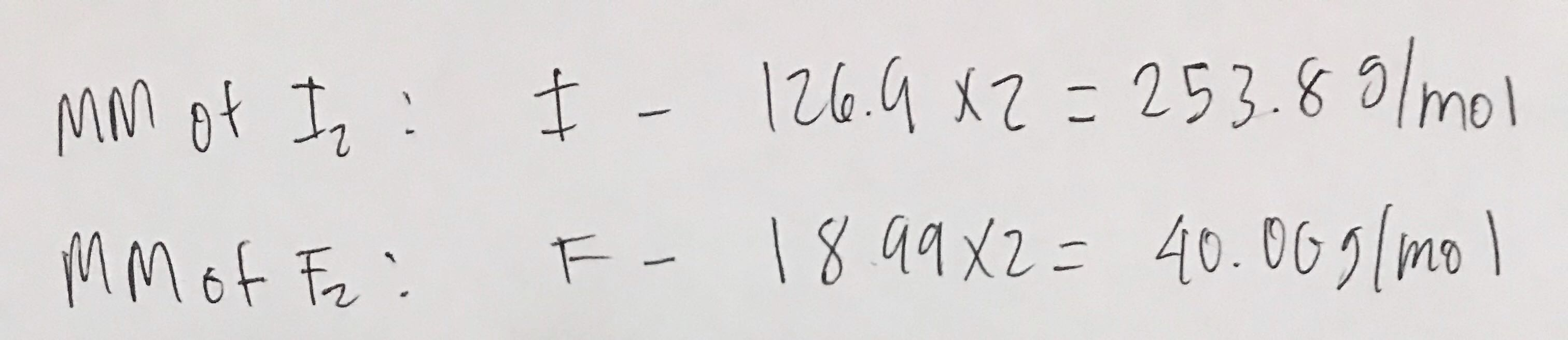# Problem: Gaseous iodine pentafluoride, IF5, can be prepared by the reaction of solid iodine and gaseous fluorine:I2 (s) + 5 F2(g) → 2 IF5(g)A 5.10-L flask containing 10.2 g I2 is charged with 10.2 g F2, and the reaction proceeds until one of the reagents is completely consumed. After the reaction is complete, the temperature in the flask is 125 oC.What is the mole fraction of IF5 in the flask?

###### FREE Expert Solution

First step is to get the molar masses of each reactant:Next step is to calculate IF5 produced from each reactant, whichever produces the least amount of moles will be the limiting reagent.

81% (161 ratings)###### Problem Details

Gaseous iodine pentafluoride, IF5, can be prepared by the reaction of solid iodine and gaseous fluorine:
I2 (s) + 5 F2(g) → 2 IF5(g)
A 5.10-L flask containing 10.2 g I2 is charged with 10.2 g F2, and the reaction proceeds until one of the reagents is completely consumed. After the reaction is complete, the temperature in the flask is 125 oC.

What is the mole fraction of IF5 in the flask?